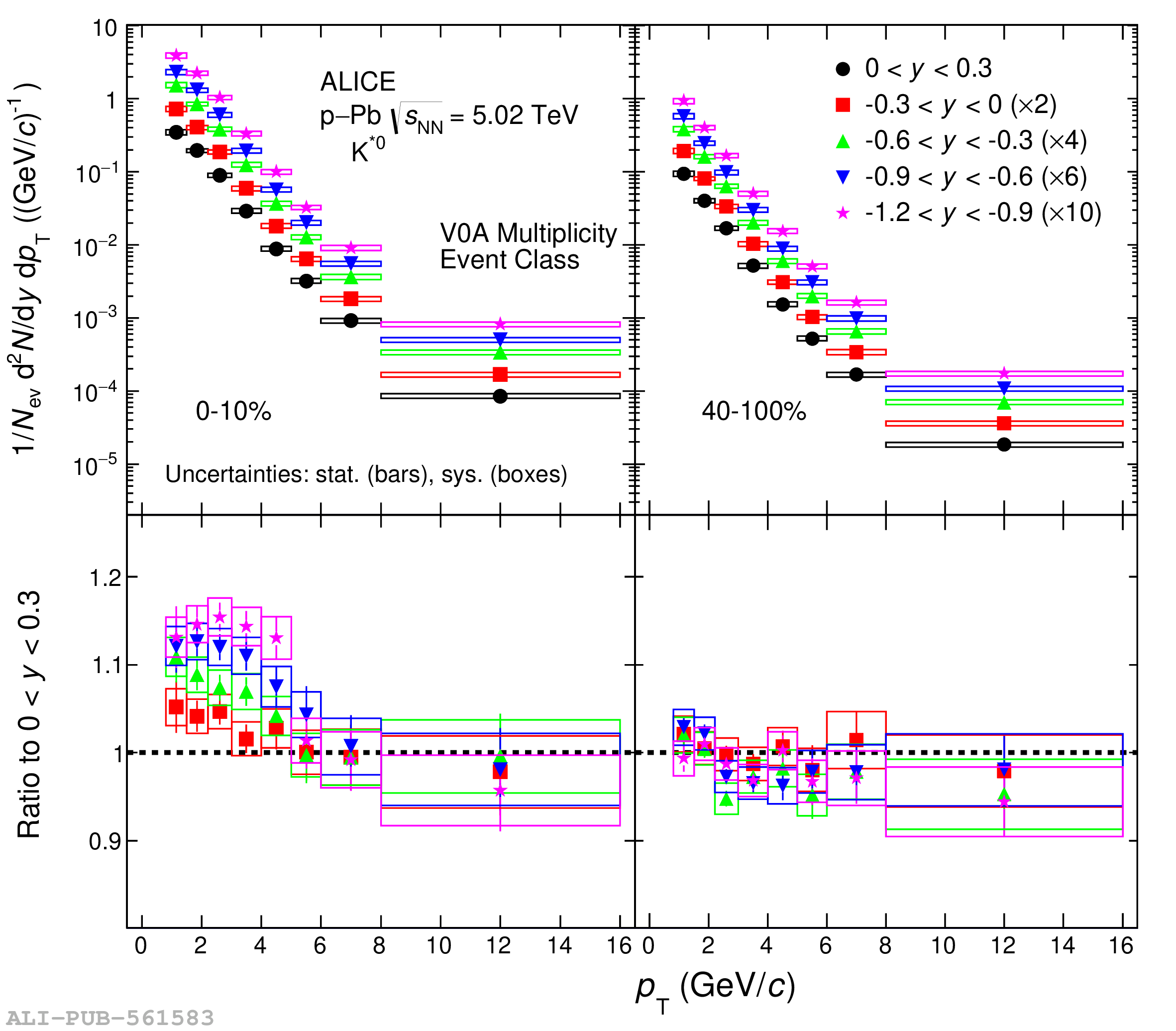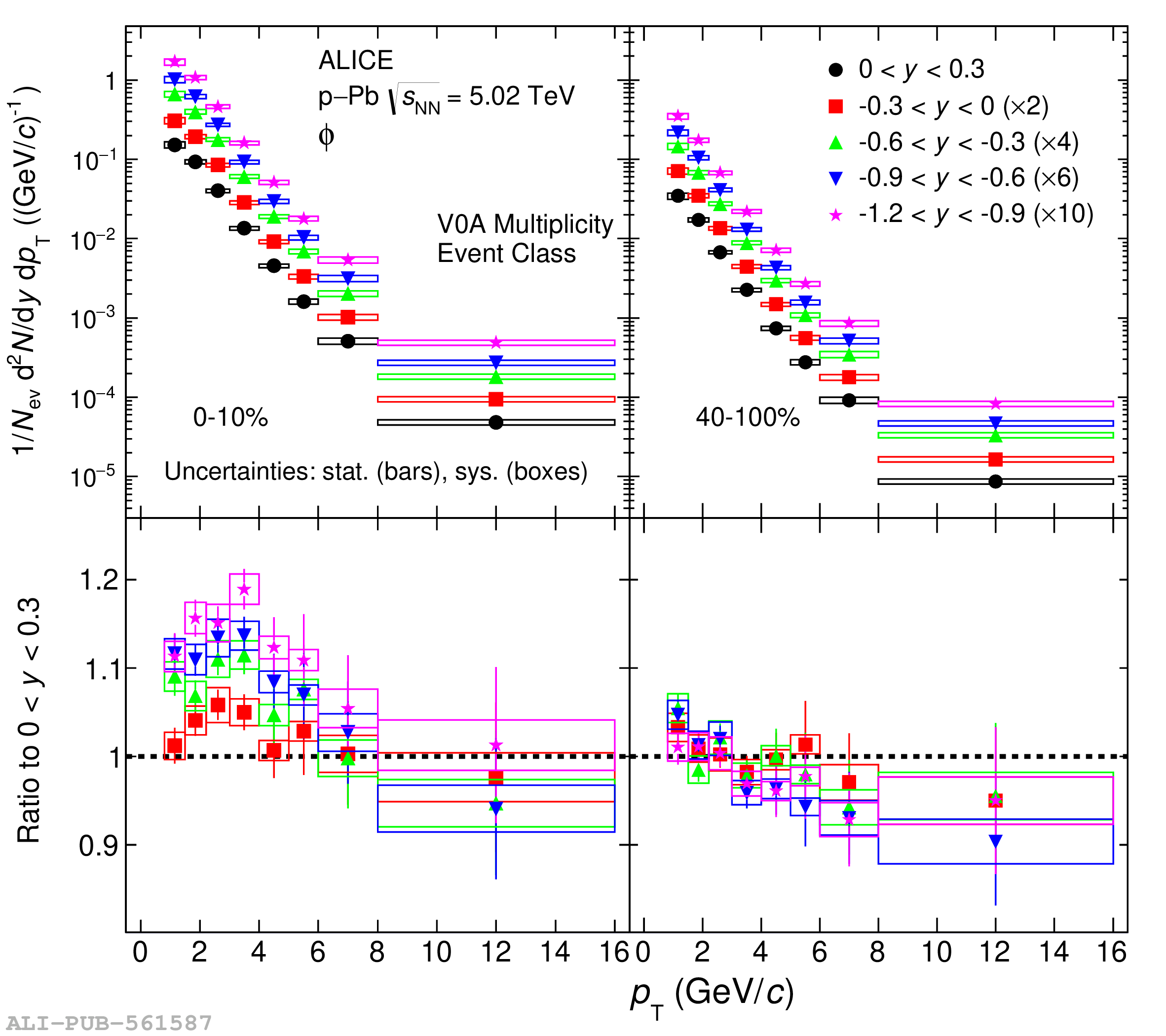# Figure 2

 Top panels: The transverse momentum spectra of \kstar (left) and \phim (right) mesons for five rapidity intervals within $-$1.2 $< y <$ 0.3 and for two multiplicity classes (0--10$\%$, 40--100$\%$) in \pPb collisions at \mbox{\snn = 5.02 TeV}. The data for different rapidity intervals are scaled for better visibility. Bottom panels: The ratios of the \pt spectra in various rapidity intervals to that in the interval 0 $< y <$ 0.3 for a given multiplicity class. The statistical and systematic uncertainties are shown as bars and boxes around the data points, respectively.